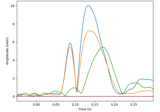# mne.inverse_sparse.gamma_map#

mne.inverse_sparse.gamma_map(evoked, forward, noise_cov, alpha, loose='auto', depth=0.8, xyz_same_gamma=True, maxit=10000, tol=1e-06, update_mode=1, gammas=None, pca=True, return_residual=False, return_as_dipoles=False, rank=None, pick_ori=None, verbose=None)[source]#

Hierarchical Bayes (Gamma-MAP) sparse source localization method.

Models each source time course using a zero-mean Gaussian prior with an unknown variance (gamma) parameter. During estimation, most gammas are driven to zero, resulting in a sparse source estimate, as in 1 and 2.

For fixed-orientation forward operators, a separate gamma is used for each source time course, while for free-orientation forward operators, the same gamma is used for the three source time courses at each source space point (separate gammas can be used in this case by using xyz_same_gamma=False).

Parameters
evokedinstance of `Evoked`

Evoked data to invert.

forward`dict`

Forward operator.

noise_covinstance of `Covariance`

Noise covariance to compute whitener.

alpha`float`

Regularization parameter (noise variance).

loose`float` | ‘auto’ | `dict`

Value that weights the source variances of the dipole components that are parallel (tangential) to the cortical surface. Can be:

• float between 0 and 1 (inclusive)

If 0, then the solution is computed with fixed orientation. If 1, it corresponds to free orientations.

• `'auto'` (default)

Uses 0.2 for surface source spaces (unless `fixed` is True) and 1.0 for other source spaces (volume or mixed).

• dict

Mapping from the key for a given source space type (surface, volume, discrete) to the loose value. Useful mostly for mixed source spaces.

depth

How to weight (or normalize) the forward using a depth prior. If float (default 0.8), it acts as the depth weighting exponent (`exp`) to use None is equivalent to 0, meaning no depth weighting is performed. It can also be a `dict` containing keyword arguments to pass to `mne.forward.compute_depth_prior()` (see docstring for details and defaults). This is effectively ignored when `method='eLORETA'`.

Changed in version 0.20: Depth bias ignored for `method='eLORETA'`.

xyz_same_gamma`bool`

Use same gamma for xyz current components at each source space point. Recommended for free-orientation forward solutions.

maxit`int`

Maximum number of iterations.

tol`float`

Tolerance parameter for convergence.

update_mode`int`

Update mode, 1: MacKay update (default), 2: Modified MacKay update.

gammas`array`, shape=(n_sources,)

Initial values for posterior variances (gammas). If None, a variance of 1.0 is used.

pca`bool`

If True the rank of the data is reduced to the true dimension.

return_residual`bool`

If True, the residual is returned as an Evoked instance.

return_as_dipoles`bool`

If True, the sources are returned as a list of Dipole instances.

rank`None` | ‘info’ | ‘full’ | `dict`

This controls the rank computation that can be read from the measurement info or estimated from the data. When a noise covariance is used for whitening, this should reflect the rank of that covariance, otherwise amplification of noise components can occur in whitening (e.g., often during source localization).

`None`

The rank will be estimated from the data after proper scaling of different channel types.

`'info'`

The rank is inferred from `info`. If data have been processed with Maxwell filtering, the Maxwell filtering header is used. Otherwise, the channel counts themselves are used. In both cases, the number of projectors is subtracted from the (effective) number of channels in the data. For example, if Maxwell filtering reduces the rank to 68, with two projectors the returned value will be 66.

`'full'`

The rank is assumed to be full, i.e. equal to the number of good channels. If a `Covariance` is passed, this can make sense if it has been (possibly improperly) regularized without taking into account the true data rank.

`dict`

Calculate the rank only for a subset of channel types, and explicitly specify the rank for the remaining channel types. This can be extremely useful if you already know the rank of (part of) your data, for instance in case you have calculated it earlier.

This parameter must be a dictionary whose keys correspond to channel types in the data (e.g. `'meg'`, `'mag'`, `'grad'`, `'eeg'`), and whose values are integers representing the respective ranks. For example, `{'mag': 90, 'eeg': 45}` will assume a rank of `90` and `45` for magnetometer data and EEG data, respectively.

The ranks for all channel types present in the data, but not specified in the dictionary will be estimated empirically. That is, if you passed a dataset containing magnetometer, gradiometer, and EEG data together with the dictionary from the previous example, only the gradiometer rank would be determined, while the specified magnetometer and EEG ranks would be taken for granted.

The default is `None`.

New in v0.18.

pick_ori`None` | “normal” | “vector”

Options:

• `None`

Pooling is performed by taking the norm of loose/free orientations. In case of a fixed source space no norm is computed leading to signed source activity.

• `"normal"`

Only the normal to the cortical surface is kept. This is only implemented when working with loose orientations.

• `"vector"`

No pooling of the orientations is done, and the vector result will be returned in the form of a `mne.VectorSourceEstimate` object.

verbose

Control verbosity of the logging output. If `None`, use the default verbosity level. See the logging documentation and `mne.verbose()` for details. Should only be passed as a keyword argument.

Returns
stcinstance of `SourceEstimate`

Source time courses.

residualinstance of `Evoked`

The residual a.k.a. data not explained by the sources. Only returned if return_residual is True.

References

1

David P. Wipf, Rey Ramírez, Jason Palmer, Scott Makeig, and Bhaskar D. Rao. Analysis of empirical bayesian methods for neuroelectromagnetic source localization. In Bernhard Schölkopf, John C. Platt, and T. Hoffman, editors, Advances in Neural Information Processing Systems 19, 1505–1512. MIT Press, 2007. URL: http://papers.nips.cc/paper/3089-analysis-of-empirical-bayesian-methods-for-neuroelectromagnetic-source-localization.pdf.

2

David Wipf and Srikantan Nagarajan. A unified Bayesian framework for MEG/EEG source imaging. NeuroImage, 44(3):947–966, 2009. doi:10.1016/j.neuroimage.2008.02.059.

## Examples using `mne.inverse_sparse.gamma_map`#Compute a sparse inverse solution using the Gamma-MAP empirical Bayesian method

Compute a sparse inverse solution using the Gamma-MAP empirical Bayesian method